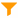# Filter

 Block Group: Table Operations Icon:The Filter block returns a new table that contains only the rows from the input table that meet a condition.

For information on using dataflow blocks, see Dataflow.

For answers to some common questions about working with tables, see Tables.

## Input/Output Properties

The following properties of the Filter block can take input and give output.

• input (table)
• condition (string)

input receives the table that you want to filter.

condition specifies the condition of the filter. Use JavaScript notation.

## Output Properties

The following properties of the Filter block can give output but cannot take input.

• print (string)
• output (table)

print returns output from the condition field. Use it for debugging.

output returns the filtered table.

## Basic Examples of the Condition Property

These examples of values for the condition property use the following table:

```row,value
0,string
1,STRING
2,StRiNg```

Using the table above, the following numeric expressions are example values for the condition property:

• `row == 0` causes row 0 to be returned.
• `row > 0` causes rows 1 and 2 to be returned.
• `row > -1` causes all rows to be returned.

Using the table above, the following string expressions are example values for the condition property:

• `String(value) == "string"` causes row 0 to be returned.
• `String(value).indexOf("S") > -1` causes rows 1 and 2 to be returned, because a capital S is included in the string in those rows.
• `String(value).toLowerCase().indexOf("string") > -1` returns all rows, because the strings are converted to lowercase before being tested.

### How to Limit a Date Range

The following example limits a table to include only rows for which the timestamp is on June 14th or June 15th, 2016.

`\$thisRow['timestamp'] > '2016-06-14' && \$thisRow['timestamp'] <= '2016-06-15T23:59:59'`

### Storing Temporary Values

You can use `\$.<variable>` in Column Mapping and Filter to store any temporary variable between rows.

The following condition returns a table that contains rows from the input table only if the v1 value in this row matches the v1 value in the previous row.

`v1==function(){var prev = \$.v1cache; \$.v1cache = v1; return prev}()`

## Example of the Filter Block

The following image shows an example of the Filter block. In this example, the table is filtered to contain only rows where the Fan_Status column holds the string OFF.